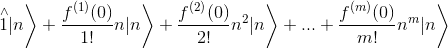# Quantum Mechanics, Simple harmonic oscillator, partition function

## Homework Statement

Compute the partition function Z = Tr(Exp(-βH)) and then the average number of particles
in a quantum state <nα > for an assembly of identical simple harmonic oscillators. The Hamiltonian is:

H = $\sum _{k}$[(nk+1/2)$\hbar - \mu$ nk]

with nk=ak+ak.

Do the calculations once for bosons and once for fermions.

## The Attempt at a Solution

For Bosons

$Z=\text{Tr}[\text{Exp}[-\text{\beta H}]]=\sum _n \langle n|\text{Exp}[-\text{\beta H}]|n\rangle =\sum _n \left\langle n\left|\text{Exp}\left[-\beta \left(\sum _k \left(n_k+\frac{1}{2}\right)\hbar -\text{\mu n}_k\right)\right]\right|n\right\rangle =$

$=\sum _n \left\langle n\left|\text{Exp}\left[-\beta \left(\sum _k (\hbar -\mu )a_k{}^+a_k-\frac{1}{2}\hbar \right)\right]\right|n\right\rangle$

I don't know how to go on from here.
Particularly I don't know how to deal with the sum in the exponential.

vela
Staff Emeritus
Homework Helper
What are the eigenstates of the Hamiltonian? I think if you understand that, evaluating the exponential should be straightforward. The sum over k is just summing over the individual harmonic oscillators.

ok, I think that I can find energy eigenstates in this way:
$\left.\left.H|n\rangle =E_n\right|n\right\rangle$

$\left.\left.\left.\left.\left(\sum _k (\hbar -\mu )a_k{}^+a_k-\frac{1}{2}\hbar \right)\right|n\right\rangle =E_n\right|n\right\rangle$

$\left.\left. \left.\left.\left.\left.\left.\left.\left.\left.(\hbar -\mu )a_1{}^+a_1\right|n\right\rangle -\frac{1}{2}\hbar \right|n\right\rangle +...+(\hbar -\mu )a_k{}^+a_k\right|n\right\rangle -\frac{1}{2}\hbar \right|n\right\rangle=E_n\right|n\right\rangle$

$\left.\left.\left.\left.\left(k\left((\hbar -\mu )n-\frac{1}{2}\hbar \right)\right)\right|n\right\rangle =E_n\right|n\right\rangle$

$E_n=\left(k\left((\hbar -\mu )n-\frac{1}{2}\hbar \right)\right)$
?

vela
Staff Emeritus
Homework Helper
Put aside the math for now. Describe in words what the eigenstates of the system are. For example, the ground state would be when all of the oscillators are in the ground state. What would the first excited state(s) be? And so on.

The first excited state is when one of the oscillators is in the E1 state,
the second excited state is when one of the oscillator is in the E2 state or
one is in E1 state and some other is also in E1 state ... etc

Isn't the answer to that question in how many ways I can distribute En energy quanta between N oscillators ?

vela
Staff Emeritus
Homework Helper
The first excited state is when one of the oscillators is in the E1 state,
So these states would be something like $\vert 1,0,0,...,0 \rangle$, $\vert 0,1,0,...,0 \rangle$, and so on, where the k-th number is n for the k-th oscillator. In other words, the eigenstates can be labeled using the n's for the individual oscillators. Do you see now how you'd evaluate the matrix elements now?
the second excited state is when one of the oscillator is in the E2 state or
one is in E1 state and some other is also in E1 state ... etc

Isn't the answer to that question in how many ways I can distribute En energy quanta between N oscillators ?

sorry , I still don't know how to do it, can you give me a more explicit hint ?

Mute
Homework Helper
You will find it more useful to leave the operator written as $\hat{n}_k$, as you are acting on the eigenstates of this operator with a function of that operator: $f(\hat{n}_k)| n \rangle$. This is actually quite easy to evaluate. Consider what happens if the function f can be expanded as a Taylor series (which is really the definition of a function of an operator). What does

$$\left(\hat{1} + \sum_{m=1}^\infty \frac{f^{(m)}(0)}{m!}\hat{n}_k^m\right)|n \rangle$$
evaluate to?

Once you understand this, making the connection to vela's hint will hopefully be clear.

Also, note that $\hbar$ should have a frequency $\omega$ attached to it: $\mathcal H = \sum_k [(\hat{n}_k + 1/2)\hbar\omega + \mu \hat{n}_k]. I think the above expression evaluates to: [itex]\left.\left.\left.\left.\overset{\wedge }{1}|n\right\rangle +\frac{f^{(1)}(0)}{1!}\overset{\wedge }{n_k}{}^1|n\right\rangle +\frac{f^{(2)}(0)}{2!}\overset{\wedge }{n_k}{}^2|n\right\rangle +\text{...}+\frac{f^{(m)}(0)}{m!}\overset{\wedge }{n_k}{}^m|n\right\rangle$ =$\left.\left(\overset{\wedge }{1}+ \sum _{m=1}^{\infty } \frac{f^{(m)}(0)}{m!}n^m\right)|n\right\rangle$

Mute
Homework Helper
I think the above expression evaluates to:

$\left.\left.\left.\left.\overset{\wedge }{1}|n\right\rangle +\frac{f^{(1)}(0)}{1!}\overset{\wedge }{n_k}{}^1|n\right\rangle +\frac{f^{(2)}(0)}{2!}\overset{\wedge }{n_k}{}^2|n\right\rangle +\text{...}+\frac{f^{(m)}(0)}{m!}\overset{\wedge }{n_k}{}^m|n\right\rangle$ =

[URL]http://latex.codecogs.com/gif.latex?\left.\left.\left.\left.\overset{\wedge%20}{1}|n\right\rangle%20+\frac{f^{(1)}(0)}{1!}n|n\right\rangle%20+\frac{f^{(2)}(0)}{2!}n^2|n\right\rangle%20+\text{...}+\frac{f^{(m)}(0)}{m!}n^m|n\right\rangle[/URL]

$\left.\left(\overset{\wedge }{1}+ \sum _{m=1}^{\infty } \frac{f^{(m)}(0)}{m!}n^m\right)|n\right\rangle$

The last line is correct; the first couple of lines should really indicate that there are infinitely many terms.

Anyways, as for the last line, you hopefully realize that that is again just the Taylor series for the function f, where the eigenvalue of $\hat{n}_k$ acting on the eigenstate $|n\rangle$ has replaced the operator! i.e.,

$$f(\hat{n})|n\rangle = f(n)|n\rangle$$
What this means is that any time you have a function of an operator that acts on an eigenstate of that operator, you can replace the operator with its eigenvalue in the function. (Caveat: it must be valid to expand the function as a Taylor series).

So, can you see how this relates to vela's hint?

P.S. - to get the hats on top of the symbols in Latex, use \hat{n}. Also, for the angle brackets, if you're going to use \left and \right on them, I would also do it for the | part. Otherwise the bras and kets look very awkward. Generally, when dealing with bras and kets I tend not to even use \left and \right, as I don't really view them as enclosing anything.

Last edited by a moderator:
vela
Staff Emeritus
$$\hat{H} = [\hbar\omega(\hat{n}_1+1/2) - \mu \hat{n}_1] + [\hbar\omega(\hat{n}_2+1/2) - \mu \hat{n}_2]$$
and the eigenstates are $|0,0\rangle$, $|1,0\rangle$, $|0,1\rangle$, $|1,1\rangle$, $|2,0\rangle$, $|0,2\rangle$, .... What do you get when $\hat{H}$ acts on each of these states?